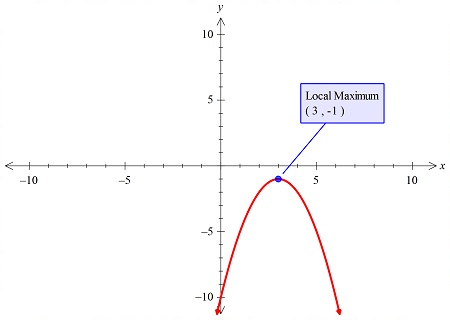# The graph of the function has one relative extreme point. Plot this point and check the concavity...

## Question:

The graph of the function has one relative extreme point. Plot this point and check the concavity there. Using only this information, sketch the graph.

{eq}f(x) = -x^2 + 6x - 10 {/eq}

## Graphs:

The graph of the parabola can be either concave upward or concave downward, and this could be easily found by the use of the graph or the calculus. Also, we can use the leading coefficient sign of the equation to check that.

## Answer and Explanation:

The graph of the function is plotted below:The grah is concave downward.

#### Learn more about this topic:Graphs: Types, Examples & Functions

from High School Algebra II: Help and Review

Chapter 16 / Lesson 11
355K